Courses

# RD Sharma Solutions - Ex-11.1,Percentage, Class 7, Math Class 7 Notes | EduRev

## RD Sharma Solutions for Class 7 Mathematics

Created by: Abhishek Kapoor

## Class 7 : RD Sharma Solutions - Ex-11.1,Percentage, Class 7, Math Class 7 Notes | EduRev

The document RD Sharma Solutions - Ex-11.1,Percentage, Class 7, Math Class 7 Notes | EduRev is a part of the Class 7 Course RD Sharma Solutions for Class 7 Mathematics.
All you need of Class 7 at this link: Class 7

#### Question 1:

Express each of the following per cents as fractions in the simplest forms:
(i) 45%
(ii) 0.25%
(iii) 150%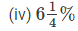(i) 45% =  45/100 = 9/20
(ii) 0.25% =  0.25/100 = 25/10000 = 5/2000 = 1/400
(iii) 150% = 150/100 = 15/10 = 3/2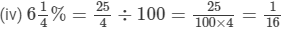#### Question 2:

Express each of the following fractions as a per cent: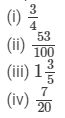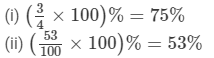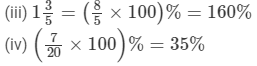97 docs

,

,

,

,

,

,

,

,

,

,

,

,

,

,

,

,

,

,

,

,

,

,

,

,

,

,

,

,

,

,

;